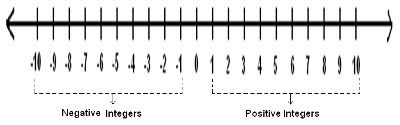Arithmetic
Associative Property
Averages
Brackets
Closure Property
Commutative Property
Conversion of Measurement Units
Cube Root
Decimal
Divisibility Principles
Equality
Exponents
Factors
Fractions
Fundamental Operations
H.C.F / G.C.D
Integers
L.C.M
Multiples
Multiplicative Identity
Multiplicative Inverse
Numbers
Percentages
Profit and Loss
Ratio and Proportion
Simple Interest
Square Root
Unitary Method
Algebra
Cartesian System
Order Relation
Polynomials
Probability
Standard Identities & their applications
Transpose
Geometry
Basic Geometrical Terms
Circle
Curves
Angles
Define Line, Line Segment and Rays
Non-Collinear Points
Parallelogram
Rectangle
Rhombus
Square
Three dimensional object
Trapezium
Triangle
Trigonometry
Trigonometry Ratios
Data-Handling
Arithmetic Mean
Frequency Distribution Table
Graphs
Median
Mode
Range

Solved Problems
Home >> Numbers >> Number line >> Integers on Number Line >>

## Integers on Number Line

 Addition on the Number line Subtraction on the Number line Multiplication on the Number line Integers on Number Line Addition of Integers on Number Line Subtraction of Integers on Number Line Compare Natural Numbers on Number Line Compare Integers on Number LineThe above figure represents Integers on number line. Numbers which are on the right side of Zero are referred as Positive Integers. Numbers which are on the Left side of Zero are referred as Negative Integers.